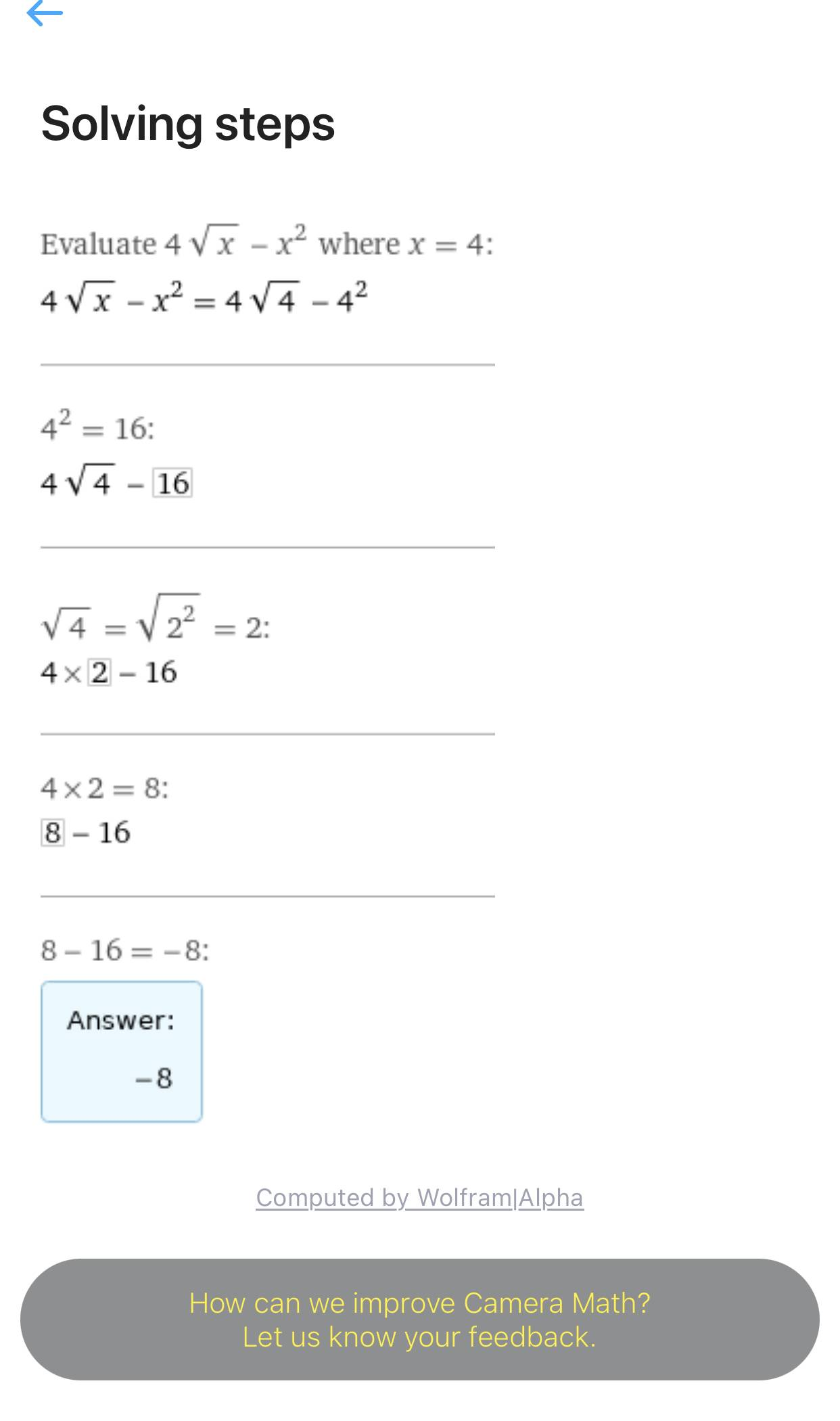# -apc.4.1.2 Find the slope at x=4

• MHB
Gold Member
MHB
$\tiny{apc.4.1.2}$
Find the slope of the tangent line to the graph of
$f(x)=-x^2+4\sqrt{x}$ at $x=4$
$a.\ 8\quad b.\ -10\quad c.\ -9\quad d.\ -5\quad e.\ -7$

$f'(x)=-2x+\frac{2}{\sqrt{x}}$
$m=f'(4)=-2(4)+\frac{2}{\sqrt{4}}=-7$ which is (e)

however not asked for here but I forgot how to find $b$ of $y=-7x+b$

Last edited:

skeeter
$y - f(4) = -7(x-4)$

clean it up ...

Gold Member
MHB
$y - f(4) = -7(x-4)$

clean it up ...

that didn't seem to be a tangent line to f{x}
$-8=-7(4)+b$
$-8+28=20=b$

$y=-7x+20$

Last edited:
skeeter
the equation in my previous post is in point-slope form, $y - y_1 = m(x - x_1)$ where $m = -7, \, x_1 = 4 \text{ and } y_1 = f(4)$

$f(4) = -8$

$y + 8 = -7(x - 4) \implies y = -7x + 20$

HOI
that didn't seem to be a tangent line to f{x}
$-8=-7(4)+b$
$-8+28=20=b$

$y=-7x+20$
Yes, it is. Since $f(x)= -x^2+ 4\sqrt{x}$ so $f(4)= -4^2+4\sqrt{4}= -16+ 8= -8$. That is, the expression skeeter gave, $y- f(4)= -7(x- 4)$ becomes $y- (-8)= y+ 8= -7x+ 28$ so, subtracting 8 from both sides, $y= -7x+ 20$ as you have.

TiffanyBK
Is the option wrong? I used cameramath and the answer is -8HOI
Is the option wrong? I used cameramath and the answer is -8

View attachment 10874
The answer to what question? You want to find the equation of the tangent line to $f(x)= -x^2+ 4\sqrt{x}$ at x= 4. You have already determined that the derivative of f, so the slope of the tangent line, at x= 4 is -7. Thus you know that the equation of the tangent line is y= -7x+ b for some number, b. So what is the value of y at x= 4? $y= f(4)= -(4^2)+ 4\sqrt{4}= -16+ 4(2)= -8$ -8 is the value of f(4). But that is not necessarily b! Now we know that we must have y= -7(4)+ b= -28+ b= -8. Adding 28 to both sides, b= 20.

The equation of the tangent line at x= 4 is y= -7x+ 20.

Last edited:
Gold Member
MHB
well i think the confusion was f(4) vs f'(4)